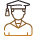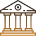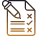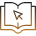# NSEJS Physics Paper: Analysis, Reference Materials And Tips

###### BySafeer PP###### Synopsis

The National Standard Examination In Junior Science (NSEJS) is a competitive exam that can be attempted by students who are in Class 10 or lower. Students whose date of birth is between January 1, 2008 and December 31, 2009 can attempt the exam this year. In this article, Careers360 analyzes the Physics section of the NSEJS. Read on to know the important physics topics from NSEJS syllabus###### Synopsis

The National Standard Examination In Junior Science (NSEJS) is a competitive exam that can be attempted by students who are in Class 10 or lower. Students whose date of birth is between January 1, 2008 and December 31, 2009 can attempt the exam this year. In this article, Careers360 analyzes the Physics section of the NSEJS. Read on to know the important physics topics from NSEJS syllabus

The National Standard Examination In Junior Science(NSEJS) is conducted every year. Toppers of NSEJS can attend the National olympiad and then topper students of National olympiad can attend the International Olympiad in Junior Science. The NSEJS syllabus and eligibility are available on their official website-https://www.iapt.org.in. The NSEJS syllabus is mainly based on the Class 9 and 10 CBSE Science.

Usually Mathematics is included in the NSEJS exam. This year's brochure does not mention Mathematics. Only Physics, Chemistry and Biology with equal weightage.

A total of 60 questions will be asked. Part A has 48 objective questions with one correct answer. These 48 questions will carry three marks and one negative mark will be awarded for wrong answers. Part B has 12 questions with more than one correct answer. This will carry six marks for the correct answer. To get the credit of six all the correct options should be marked. No negative marking for six mark questions.

## Physics NSEJS Mark Distribution

A total of six NSEJS previous year papers are analysed. The Physics part of NSEJS is divided into 11 chapters. Some basic mathematics questions are in the physics part based on linear graphs or equations of the straight line. This is included as the first chapter in the analysis. See the chapterwise mark distribution of NSEJS Physics below.

NSEJS Physics Syllabus Chapter wise Weightage

 Chapter Total Mark Basic Mathematics 6 Electricity 72 Force and Laws of Motion 15 Gravitation 36 Human Eye and Colourful World 3 Light Reflection and Refraction 51 Magnetic Effects of Electric Current 18 Motion 15 Sound 15 Thermal Properties of matter 15 Work and Energy 42

Based on the weightage mark the important chapters from Physics part of NSEJS syllabus are Electricity, Light, Work Energy And Power and Gravitation. For the analysis purpose questions related to oscillations and SHM are included in Work And Energy. And the Chapter Thermal Properties Of Matter can be studied using the Class 11 Physics book.

## NSEJS Study Materials

 Chapter Reference Materials Basic Mathematics Basic Mathematics Learned From Class 6 to 10. No separate reference required. Mainly focus on coordinate geometry Electricity Class 10 NCERT Science Book+Study properties of electric field and Coulomb's Law. NCERT Exemplar problems for Class 10 Science chapter Electricity Force and Laws of Motion Class 9 NCERT science book+learn to draw free body diagram and solve problems Gravitation Class 9 NCERT Science book+Study Archimedes principle Human Eye and Colourful World Class 10 NCERT Physics book+Exemplar problems Light Reflection and Refraction NCERT Class 10 Science book+Exemplar problems Magnetic Effects of Electric Current NCERT Class 10 Science book chapter 13 Motion Class 9 NCERT Science book Sound Class 9 NCERT Science book Thermal Properties of matter NCERTClass 11 Physics book vol 2 chapter 11 Work and Energy Class 9 NCERT Science book+concept of oscillations and simple pendulum(Refer to Class 11 NCERT chapter Oscillations)

## Tips To Prepare NSEJS Physics

• Focus on theory and problems of NCERT.

• Understand all the derivations.

• Solve NSEJS question papers. The NSEJS papers are available at the official website of Indian Association of Physics Teachers.

• Solve NCERT exemplar problems of Class 9 and 10 Science.

• Focus on miscellaneous topics like Pressure Inside A Liquid, Archimedes Principle, Oscillations, Simple Pendulum, Thermal Properties Of Matter, Properties Of Electric Field And Coulomb's Law.

• From Electricity chapter solve more circuits.

• Study all the chapters of Class 9 and 10 NCERT Physics part of the Science book.

• Science
• Nature
• Physical sciences
• Physics
• Central Board of Secondary Education
• Mathematics
• Applied and interdisciplinary physics
• Mechanics
• Newton's laws of motion
• National Standard Examination in Junior Science

## Careers360 helping shape your Career for a better tomorrow#### 250M+

Students#### 30,000+

Colleges#### 500+

ExamsE-Books